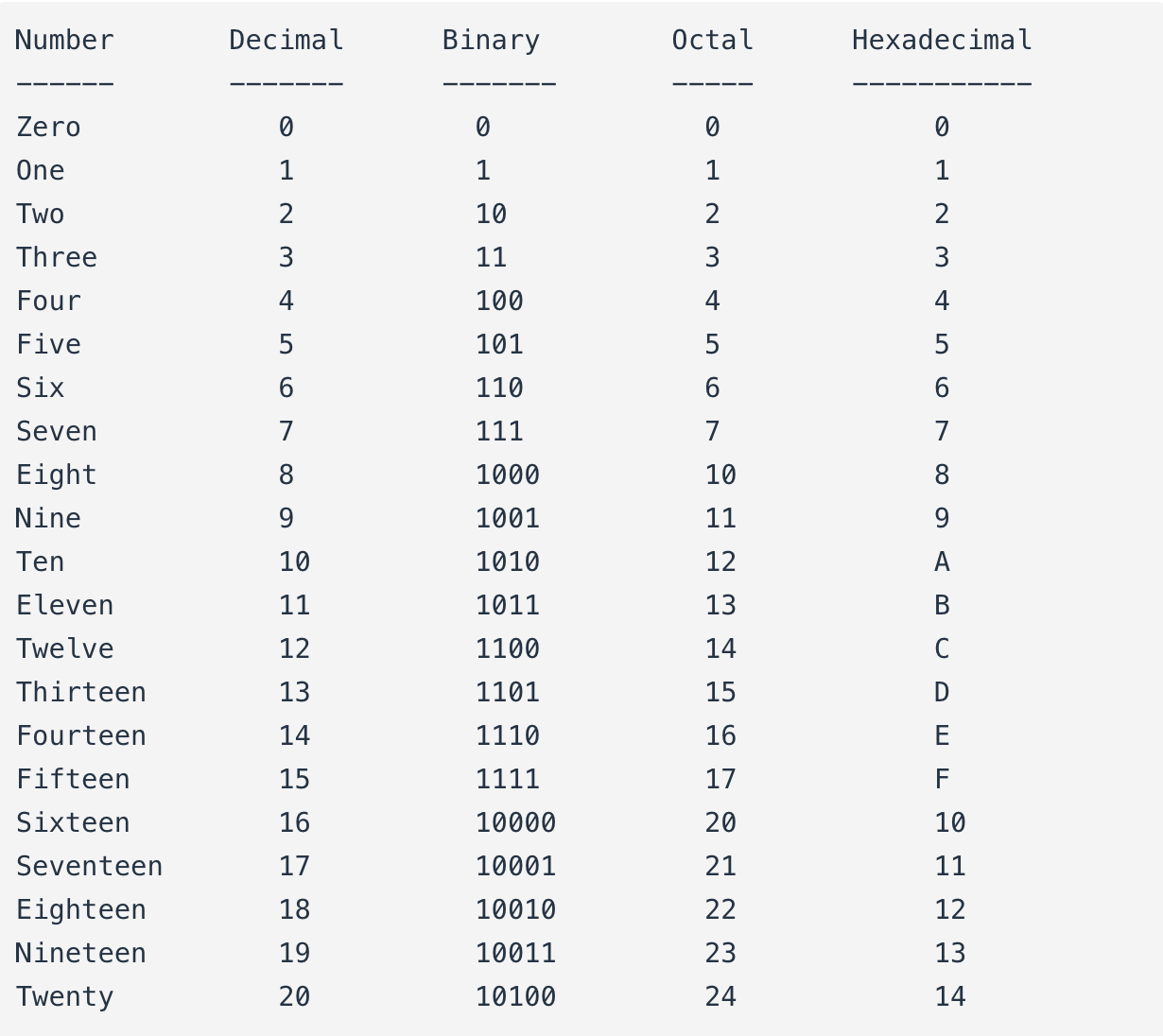## What is the difference between numbers and systems of numeration?

The essential distinction between the two is much like that between an object and the spoken word(s) we associate with it. A house is still a house regardless of whether we call it by its English name house or its Spanish name casa. The first is the actual thing, while the second is merely the symbol for the thing.

# Numbers versus Numeration

## Chapter 2 - Binary Arithmetic

It is imperative to understand that the type of numeration system used to represent numbers has no impact on the outcome of any arithmetical function (addition, subtraction, multiplication, division, roots, powers, or logarithms).

A number is a number is a number; one plus one will always equal two (so long as we’re dealing with real numbers), no matter how you symbolize one, one, and two. A prime number in decimal form is still prime if its shown in binary form, or octal, or hexadecimal. π is still the ratio between the circumference and diameter of a circle, no matter what symbol(s) you use to denote its value.

The essential functions and interrelations of mathematics are unaffected by the particular system of symbols we might choose to represent quantities. This distinction between numbers and systems of numeration is critical to understand.

The essential distinction between the two is much like that between an object and the spoken word(s) we associate with it. A house is still a house regardless of whether we call it by its English name house or its Spanish name casa. The first is the actual thing, while the second is merely the symbol for the thing.

That being said, performing a simple arithmetic operation such as addition (longhand) in binary form can be confusing to a person accustomed to working with decimal numeration only. In this lesson, we’ll explore the techniques used to perform simple arithmetic functions on binary numbers, since these techniques will be employed in the design of electronic circuits to do the same.

You might take longhand addition and subtraction for granted, having used a calculator for so long, but deep inside that calculator’s circuitry, all those operations are performed “longhand,” using binary numeration. To understand how that’s accomplished, we need to review the basics of arithmetic.

• Share
Published under the terms and conditions of the Design Science License